# PPT-Rectilinear Motion.pptx

May. 30, 2023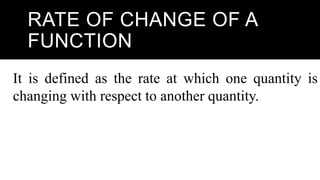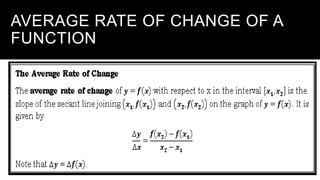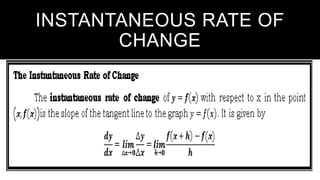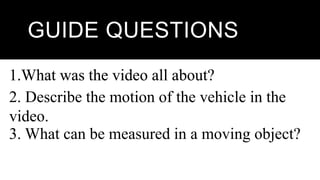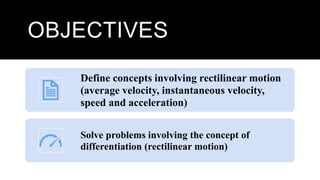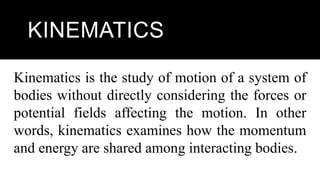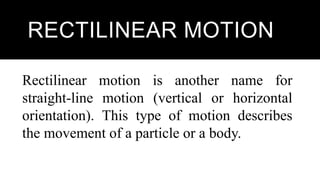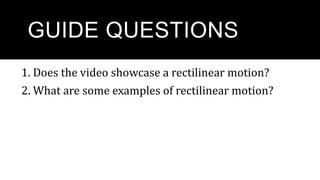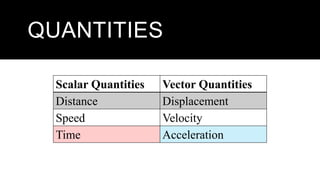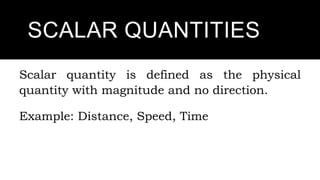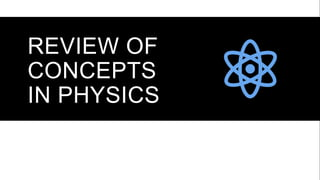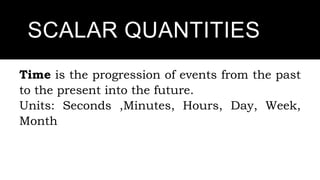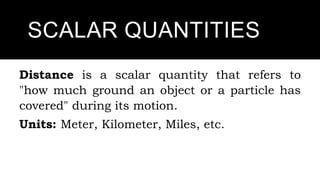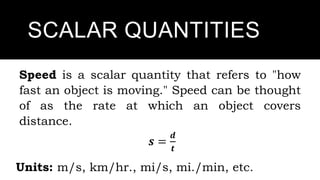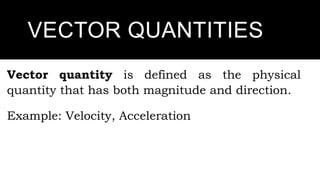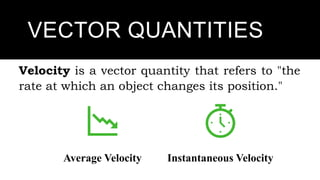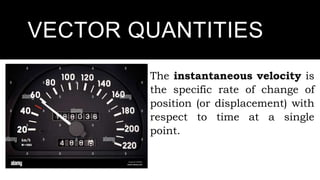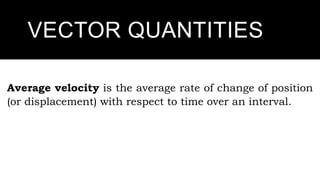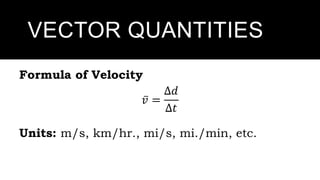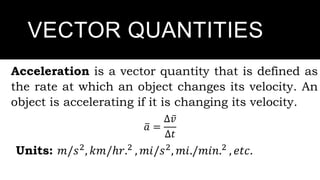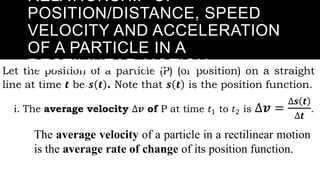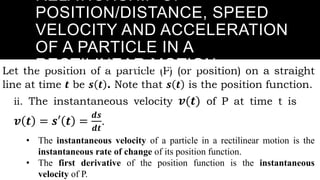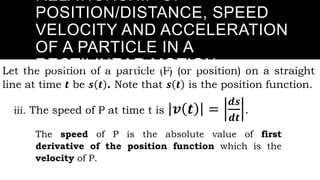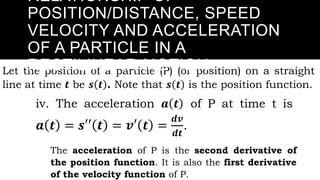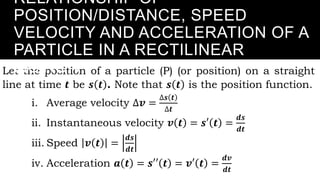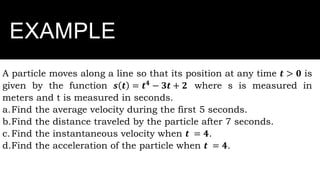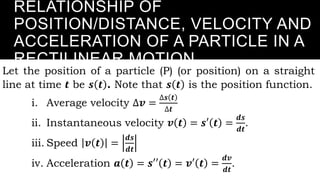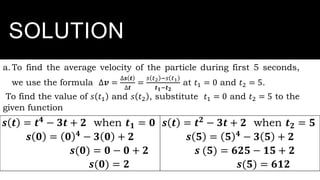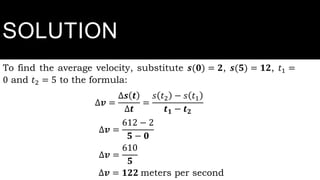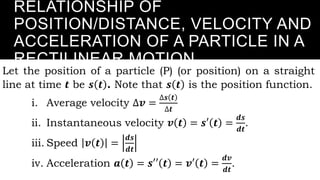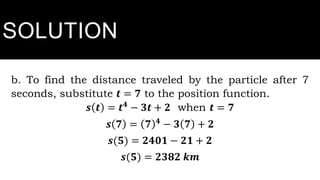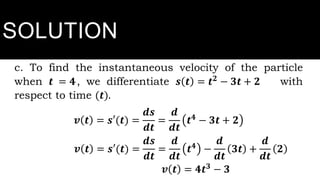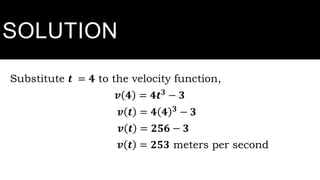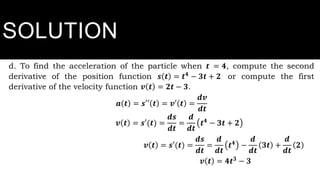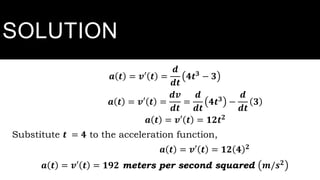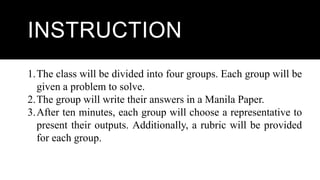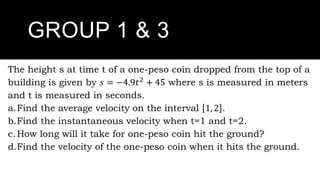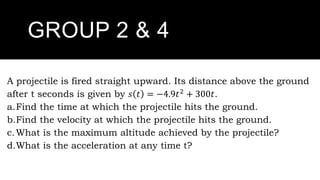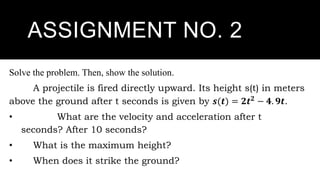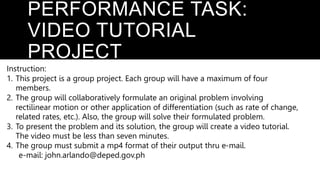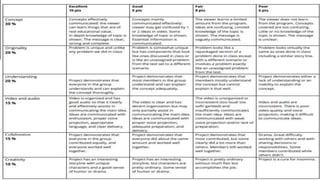1 of 47

### PPT-Rectilinear Motion.pptx

• 1. REVIEW OF THE PREVIOUS LESSON
• 2. What was the previous discussion last meeting all about?
• 3. RATE OF CHANGE OF A FUNCTION It is defined as the rate at which one quantity is changing with respect to another quantity.
• 4. AVERAGE RATE OF CHANGE OF A FUNCTION
• 5. INSTANTANEOUS RATE OF CHANGE
• 6. ACTIVITY
• 7. GUIDE QUESTIONS 1.What was the video all about? 1.What was the video all about? 1.What was the video all about? 2. Describe the motion of the vehicle in the video. 3. What can be measured in a moving object?
• 8. RECTILINEAR MOTION
• 9. OBJECTIVES Define concepts involving rectilinear motion (average velocity, instantaneous velocity, speed and acceleration) Solve problems involving the concept of differentiation (rectilinear motion)
• 10. KINEMATICS Kinematics is the study of motion of a system of bodies without directly considering the forces or potential fields affecting the motion. In other words, kinematics examines how the momentum and energy are shared among interacting bodies.
• 11. RECTILINEAR MOTION Rectilinear motion is another name for straight-line motion (vertical or horizontal orientation). This type of motion describes the movement of a particle or a body.
• 12. GUIDE QUESTIONS 1. Does the video showcase a rectilinear motion? 2. What are some examples of rectilinear motion?
• 13. QUANTITIES Scalar Quantities Vector Quantities Distance Displacement Speed Velocity Time Acceleration
• 14. SCALAR QUANTITIES Scalar quantity is defined as the physical quantity with magnitude and no direction. Example: Distance, Speed, Time
• 15. REVIEW OF CONCEPTS IN PHYSICS
• 16. SCALAR QUANTITIES Time is the progression of events from the past to the present into the future. Units: Seconds ,Minutes, Hours, Day, Week, Month
• 17. SCALAR QUANTITIES Distance is a scalar quantity that refers to "how much ground an object or a particle has covered" during its motion. Units: Meter, Kilometer, Miles, etc.
• 18. SCALAR QUANTITIES Speed is a scalar quantity that refers to "how fast an object is moving." Speed can be thought of as the rate at which an object covers distance. 𝒔 = 𝒅 𝒕 Units: m/s, km/hr., mi/s, mi./min, etc.
• 19. VECTOR QUANTITIES Vector quantity is defined as the physical quantity that has both magnitude and direction. Example: Velocity, Acceleration
• 20. VECTOR QUANTITIES Velocity is a vector quantity that refers to "the rate at which an object changes its position." Average Velocity Instantaneous Velocity
• 21. VECTOR QUANTITIES The instantaneous velocity is the specific rate of change of position (or displacement) with respect to time at a single point.
• 22. VECTOR QUANTITIES Average velocity is the average rate of change of position (or displacement) with respect to time over an interval.
• 23. VECTOR QUANTITIES Formula of Velocity 𝑣 = ∆𝑑 ∆𝑡 Units: m/s, km/hr., mi/s, mi./min, etc.
• 24. VECTOR QUANTITIES Acceleration is a vector quantity that is defined as the rate at which an object changes its velocity. An object is accelerating if it is changing its velocity. 𝑎 = ∆𝑣 ∆𝑡 Units: 𝑚/𝑠2 , 𝑘𝑚/ℎ𝑟.2 , 𝑚𝑖/𝑠2 , 𝑚𝑖./𝑚𝑖𝑛.2 , 𝑒𝑡𝑐.
• 25. Let the position of a particle (P) (or position) on a straight line at time 𝒕 be 𝒔 𝒕 . Note that 𝒔 𝒕 is the position function. i. The average velocity ∆𝒗 of P at time 𝑡1 to 𝑡2 is ∆𝒗 = ∆𝒔 𝒕 ∆𝒕 . The average velocity of a particle in a rectilinear motion is the average rate of change of its position function. RELATIONSHIP OF POSITION/DISTANCE, SPEED VELOCITY AND ACCELERATION OF A PARTICLE IN A RECTILINEAR MOTION
• 26. Let the position of a particle (P) (or position) on a straight line at time 𝒕 be 𝒔 𝒕 . Note that 𝒔 𝒕 is the position function. ii. The instantaneous velocity 𝒗(𝒕) of P at time t is 𝒗 𝒕 = 𝒔′ 𝒕 = 𝒅𝒔 𝒅𝒕 . • The instantaneous velocity of a particle in a rectilinear motion is the instantaneous rate of change of its position function. • The first derivative of the position function is the instantaneous velocity of P. RELATIONSHIP OF POSITION/DISTANCE, SPEED VELOCITY AND ACCELERATION OF A PARTICLE IN A RECTILINEAR MOTION
• 27. Let the position of a particle (P) (or position) on a straight line at time 𝒕 be 𝒔 𝒕 . Note that 𝒔 𝒕 is the position function. iii. The speed of P at time t is 𝒗 𝒕 = 𝒅𝒔 𝒅𝒕 . The speed of P is the absolute value of first derivative of the position function which is the velocity of P. RELATIONSHIP OF POSITION/DISTANCE, SPEED VELOCITY AND ACCELERATION OF A PARTICLE IN A RECTILINEAR MOTION
• 28. Let the position of a particle (P) (or position) on a straight line at time 𝒕 be 𝒔 𝒕 . Note that 𝒔 𝒕 is the position function. iv. The acceleration 𝒂 𝒕 of P at time t is 𝒂 𝒕 = 𝒔′′ 𝒕 = 𝒗′ 𝒕 = 𝒅𝒗 𝒅𝒕 . The acceleration of P is the second derivative of the position function. It is also the first derivative of the velocity function of P. RELATIONSHIP OF POSITION/DISTANCE, SPEED VELOCITY AND ACCELERATION OF A PARTICLE IN A RECTILINEAR MOTION
• 29. Let the position of a particle (P) (or position) on a straight line at time 𝒕 be 𝒔 𝒕 . Note that 𝒔 𝒕 is the position function. i. Average velocity ∆𝒗 = ∆𝒔 𝒕 ∆𝒕 ii. Instantaneous velocity 𝒗 𝒕 = 𝒔′ 𝒕 = 𝒅𝒔 𝒅𝒕 iii. Speed 𝒗 𝒕 = 𝒅𝒔 𝒅𝒕 iv. Acceleration 𝒂 𝒕 = 𝒔′′ 𝒕 = 𝒗′ 𝒕 = 𝒅𝒗 𝒅𝒕 RELATIONSHIP OF POSITION/DISTANCE, SPEED VELOCITY AND ACCELERATION OF A PARTICLE IN A RECTILINEAR MOTION
• 30. EXAMPLE A particle moves along a line so that its position at any time 𝒕 > 𝟎 is given by the function 𝒔 𝒕 = 𝒕𝟒 − 𝟑𝒕 + 𝟐 where s is measured in meters and t is measured in seconds. a.Find the average velocity during the first 5 seconds. b.Find the distance traveled by the particle after 7 seconds. c.Find the instantaneous velocity when 𝒕 = 𝟒. d.Find the acceleration of the particle when 𝒕 = 𝟒.
• 31. RELATIONSHIP OF POSITION/DISTANCE, VELOCITY AND ACCELERATION OF A PARTICLE IN A RECTILINEAR MOTION Let the position of a particle (P) (or position) on a straight line at time 𝒕 be 𝒔 𝒕 . Note that 𝒔 𝒕 is the position function. i. Average velocity ∆𝒗 = ∆𝒔 𝒕 ∆𝒕 ii. Instantaneous velocity 𝒗 𝒕 = 𝒔′ 𝒕 = 𝒅𝒔 𝒅𝒕 . iii. Speed 𝒗 𝒕 = 𝒅𝒔 𝒅𝒕 iv. Acceleration 𝒂 𝒕 = 𝒔′′ 𝒕 = 𝒗′ 𝒕 = 𝒅𝒗 𝒅𝒕 .
• 32. SOLUTION a.To find the average velocity of the particle during first 5 seconds, we use the formula ∆𝒗 = ∆𝒔 𝒕 ∆𝒕 = 𝑠 𝑡2 −𝑠 𝑡1 𝒕𝟏−𝒕𝟐 at 𝑡1 = 0 and 𝑡2 = 5. To find the value of 𝑠 𝑡1 and 𝑠 𝑡2 , substitute 𝑡1 = 0 and 𝑡2 = 5 to the given function 𝒔 𝒕 = 𝒕𝟒 − 𝟑𝒕 + 𝟐 when 𝒕𝟏 = 𝟎 𝒔 𝟎 = 𝟎 𝟒 − 𝟑 𝟎 + 𝟐 𝒔(𝟎) = 𝟎 − 𝟎 + 𝟐 𝒔(𝟎) = 𝟐 𝒔 𝒕 = 𝒕𝟐 − 𝟑𝒕 + 𝟐 when 𝒕𝟐 = 𝟓 𝒔 𝟓 = 𝟓 𝟒 − 𝟑 𝟓 + 𝟐 𝒔 (𝟓) = 𝟔𝟐𝟓 − 𝟏𝟓 + 𝟐 𝒔(𝟓) = 𝟔𝟏𝟐
• 33. SOLUTION To find the average velocity, substitute 𝒔(𝟎) = 𝟐, 𝒔(𝟓) = 𝟏𝟐, 𝑡1 = 0 and 𝑡2 = 5 to the formula: ∆𝒗 = ∆𝒔 𝒕 ∆𝒕 = 𝑠 𝑡2 − 𝑠 𝑡1 𝒕𝟏 − 𝒕𝟐 ∆𝒗 = 612 − 2 𝟓 − 𝟎 ∆𝒗 = 610 𝟓 ∆𝒗 = 𝟏𝟐𝟐 meters per second
• 34. RELATIONSHIP OF POSITION/DISTANCE, VELOCITY AND ACCELERATION OF A PARTICLE IN A RECTILINEAR MOTION Let the position of a particle (P) (or position) on a straight line at time 𝒕 be 𝒔 𝒕 . Note that 𝒔 𝒕 is the position function. i. Average velocity ∆𝒗 = ∆𝒔 𝒕 ∆𝒕 ii. Instantaneous velocity 𝒗 𝒕 = 𝒔′ 𝒕 = 𝒅𝒔 𝒅𝒕 . iii. Speed 𝒗 𝒕 = 𝒅𝒔 𝒅𝒕 iv. Acceleration 𝒂 𝒕 = 𝒔′′ 𝒕 = 𝒗′ 𝒕 = 𝒅𝒗 𝒅𝒕 .
• 35. SOLUTION b. To find the distance traveled by the particle after 7 seconds, substitute 𝒕 = 𝟕 to the position function. 𝒔 𝒕 = 𝒕𝟒 − 𝟑𝒕 + 𝟐 when 𝒕 = 𝟕 𝒔 𝟕 = 𝟕 𝟒 − 𝟑 𝟕 + 𝟐 𝒔(𝟓) = 𝟐𝟒𝟎𝟏 − 𝟐𝟏 + 𝟐 𝒔(𝟓) = 𝟐𝟑𝟖𝟐 𝒌𝒎
• 36. SOLUTION c. To find the instantaneous velocity of the particle when 𝒕 = 𝟒, we differentiate 𝒔 𝒕 = 𝒕𝟐 − 𝟑𝒕 + 𝟐 with respect to time (𝒕). 𝒗 𝒕 = 𝒔′(𝒕) = 𝒅𝒔 𝒅𝒕 = 𝒅 𝒅𝒕 𝒕𝟒 − 𝟑𝒕 + 𝟐 𝒗 𝒕 = 𝒔′(𝒕) = 𝒅𝒔 𝒅𝒕 = 𝒅 𝒅𝒕 𝒕𝟒 − 𝒅 𝒅𝒕 𝟑𝒕 + 𝒅 𝒅𝒕 𝟐 𝒗 𝒕 = 𝟒𝒕𝟑 − 𝟑
• 37. SOLUTION Substitute 𝒕 = 𝟒 to the velocity function, 𝒗 𝟒 = 𝟒𝒕𝟑 − 𝟑 𝒗 𝒕 = 𝟒 𝟒 𝟑 − 𝟑 𝒗 𝒕 = 𝟐𝟓𝟔 − 𝟑 𝒗 𝒕 = 𝟐𝟓𝟑 meters per second
• 38. RELATIONSHIP OF POSITION/DISTANCE, VELOCITY AND ACCELERATION OF A PARTICLE IN A RECTILINEAR MOTION Let the position of a particle (P) (or position) on a straight line at time 𝒕 be 𝒔 𝒕 . Note that 𝒔 𝒕 is the position function. i. Average velocity ∆𝒗 = ∆𝒔 𝒕 ∆𝒕 ii. Instantaneous velocity 𝒗 𝒕 = 𝒔′ 𝒕 = 𝒅𝒔 𝒅𝒕 . iii. Speed 𝒗 𝒕 = 𝒅𝒔 𝒅𝒕 iv. Acceleration 𝒂 𝒕 = 𝒔′′ 𝒕 = 𝒗′ 𝒕 = 𝒅𝒗 𝒅𝒕 .
• 39. SOLUTION d. To find the acceleration of the particle when 𝒕 = 𝟒, compute the second derivative of the position function 𝒔 𝒕 = 𝒕𝟒 − 𝟑𝒕 + 𝟐 or compute the first derivative of the velocity function 𝒗 𝒕 = 𝟐𝒕 − 𝟑. 𝒂 𝒕 = 𝒔′′ 𝒕 = 𝒗′ 𝒕 = 𝒅𝒗 𝒅𝒕 𝒗 𝒕 = 𝒔′(𝒕) = 𝒅𝒔 𝒅𝒕 = 𝒅 𝒅𝒕 𝒕𝟒 − 𝟑𝒕 + 𝟐 𝒗 𝒕 = 𝒔′(𝒕) = 𝒅𝒔 𝒅𝒕 = 𝒅 𝒅𝒕 𝒕𝟒 − 𝒅 𝒅𝒕 𝟑𝒕 + 𝒅 𝒅𝒕 𝟐 𝒗 𝒕 = 𝟒𝒕𝟑 − 𝟑
• 40. SOLUTION 𝒂 𝒕 = 𝒗′ 𝒕 = 𝒅 𝒅𝒕 𝟒𝒕𝟑 − 𝟑 𝒂 𝒕 = 𝒗′ 𝒕 = 𝒅𝒗 𝒅𝒕 = 𝒅 𝒅𝒕 𝟒𝒕𝟑 − 𝒅 𝒅𝒕 𝟑 𝒂 𝒕 = 𝒗′ 𝒕 = 𝟏𝟐𝒕𝟐 Substitute 𝒕 = 𝟒 to the acceleration function, 𝒂 𝒕 = 𝒗′ 𝒕 = 𝟏𝟐 𝟒 𝟐 𝒂 𝒕 = 𝒗′ 𝒕 = 𝟏𝟗𝟐 meters per second squared 𝒎/𝒔𝟐
• 41. GROUP ACTIVITY
• 42. INSTRUCTION 1.The class will be divided into four groups. Each group will be given a problem to solve. 2.The group will write their answers in a Manila Paper. 3.After ten minutes, each group will choose a representative to present their outputs. Additionally, a rubric will be provided for each group.
• 43. GROUP 1 & 3 The height s at time t of a one-peso coin dropped from the top of a building is given by 𝑠 = −4.9𝑡2 + 45 where s is measured in meters and t is measured in seconds. a.Find the average velocity on the interval 1, 2 . b.Find the instantaneous velocity when t=1 and t=2. c.How long will it take for one-peso coin hit the ground? d.Find the velocity of the one-peso coin when it hits the ground.
• 44. GROUP 2 & 4 A projectile is fired straight upward. Its distance above the ground after t seconds is given by 𝑠 𝑡 = −4.9𝑡2 + 300𝑡. a.Find the time at which the projectile hits the ground. b.Find the velocity at which the projectile hits the ground. c.What is the maximum altitude achieved by the projectile? d.What is the acceleration at any time t?
• 45. ASSIGNMENT NO. 2 Solve the problem. Then, show the solution. A projectile is fired directly upward. Its height s(t) in meters above the ground after t seconds is given by 𝒔(𝒕) = 𝟐𝒕𝟐 − 𝟒. 𝟗𝒕. • What are the velocity and acceleration after t seconds? After 10 seconds? • What is the maximum height? • When does it strike the ground?
• 46. PERFORMANCE TASK: VIDEO TUTORIAL PROJECT Instruction: 1. This project is a group project. Each group will have a maximum of four members. 2. The group will collaboratively formulate an original problem involving rectilinear motion or other application of differentiation (such as rate of change, related rates, etc.). Also, the group will solve their formulated problem. 3. To present the problem and its solution, the group will create a video tutorial. The video must be less than seven minutes. 4. The group must submit a mp4 format of their output thru e-mail. e-mail: john.arlando@deped.gov.ph

### Editor's Notes

1. Guide Questions: 1. Does the video showcase a rectilinear motion? 2. What are some examples of rectilinear motion?